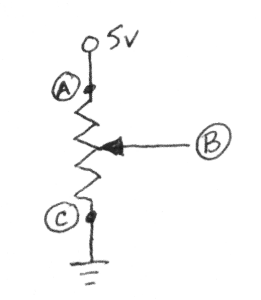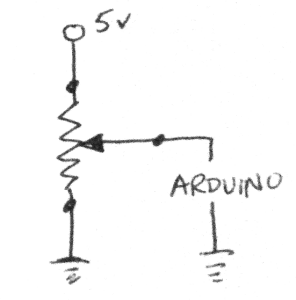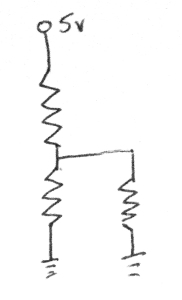# Arduino Notes: PotentiometerOther Rants and Raves

October 2009 -

The potentiometer has three leads: a,b,c.  A is on one end. C is on the other. The pot is a resister between A and C. The B lead is in the middle. When you twist the pot to one side it touches the A end; twist the other way and it touches the C end.

Lead A we attach to Vcc (5vdc). Lead C we attach to ground. The B lead is attached to an input on the Arduino. The Analog.read function measures the voltage between B and ground. When the knob is twisted so that B touches A then B is at 5v, when you twist it the other way and B touches C, then B is at 0.What is the voltage on B when the potentiometer is in the middle? To figure this out we have to consider the potentiometer as two resistors: one from A to B and one from B to C. Current flows from A (Vcc) to B and then it splits. It flows from B to C (ground) and from B through the arduino input to ground. So we have two resistive segments.Let's assume that the potentiometer is 1M ohms from A to C. Since the potentiometer in this example has set B in the middle, we know that A to B has a resistance of 500,000 ohms. The second segment has two resistors in parallel: B to C and B through the Arduino.We calculate parallel resistance as 1/ (1/r1 + 1/r2). B to C is 500,000 ohms. The Arduino input to ground is 1M ohms. So the second segment has a resistance of 333,333 ohms.

The voltage at B is split between the two segments in the ratio of their resistance. So we have two segments:

5vdc -----   500,000 ohms  ---- B ----- 333,333 ohms ------ ground

In this case B will be at 2 volts. Get that: the potentiometer is in the middle, but B is at LESS than half. That's not so good.

Let's consider 3 positions. The potentiometer at 75%, 50%, 25% of the dial. Now the B voltages are:

5vdc -----   750,000 ohms  ---- B ----- 200,000 ohms ------ ground       B is at 1 volt

5vdc -----   500,000 ohms  ---- B ----- 333,333 ohms ------ ground       B is at 2 volts

5vdc -----   250,000 ohms  ---- B ----- 428,571 ohms ------ ground       B is at 3.1 volts

The output voltage is not linear as we turn the potentiometer.

Let's do it again with a 1k pot.

5vdc -----   750 ohms  ---- B ----- 249.93 ohms ------ ground       B is at 1.25 volts

5vdc -----   500 ohms  ---- B ----- 499.75 ohms ------ ground       B is at 2.5   volts

5vdc -----   250 ohms  ---- B ----- 749.43 ohms ------ ground       B is at 3.75 volts

We see that with the low resistance potentiometer, its values dominate the calculation of resistance for the parallel segment of the circuit. As a result the voltage at B will vary in a linear fashion as the potentiometer is turned. This is a good thing.

Jim Schrempp is a sometimes freelance writer (only Vanity Press will publish his work) living in Saratoga, California. His writings have appeared on numerous pages on his own web site. The opinions expressed in this piece are those of the writer and do not necessarily represent those of anyone else (although Jim wishes more people shared his opinions)